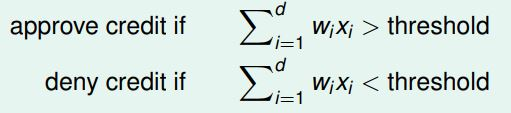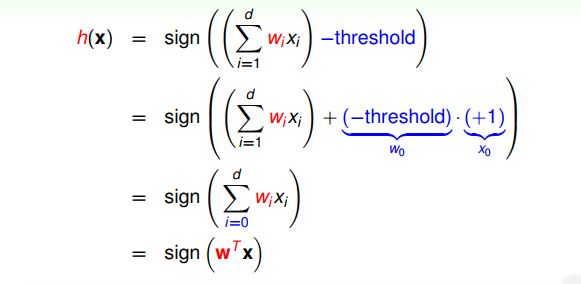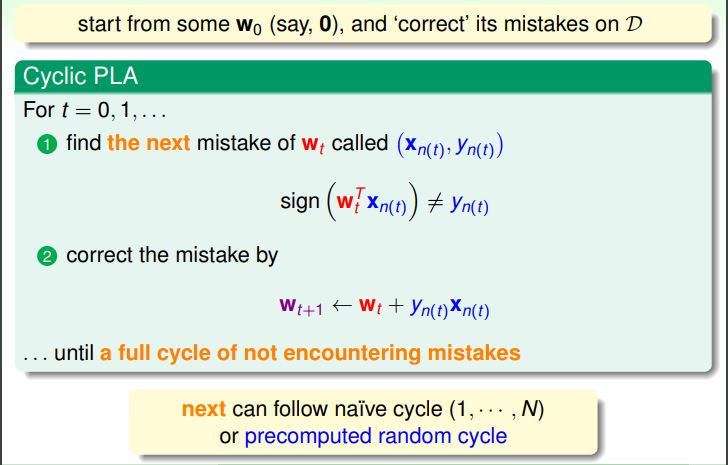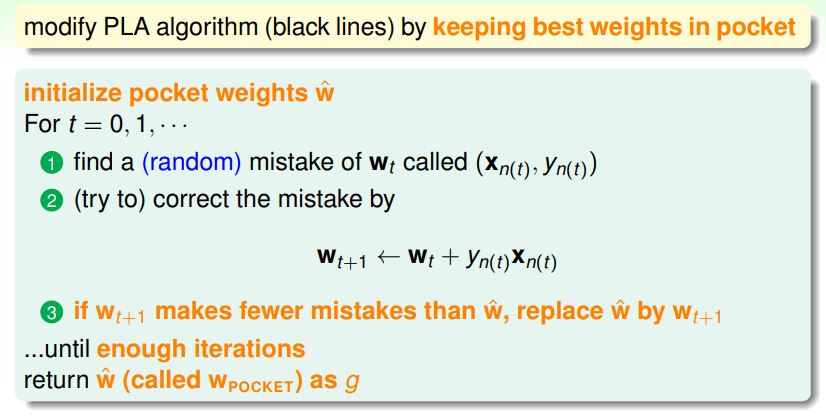# 《机器学习基石》课程笔记（2）

1. Perceptron Hypothesis Set
对于银行是否发送信用卡的问题，把每位顾客的年龄、年收入等特征看成一个向量x=(x1,x2,...xd)$\mathbf{x} = (x_1,x_2,...x_d)$，计算顾客每个特征与权重的乘积之和，如果结果大于某个阈值（threshold），那么就发送信用卡，否则不发送信用卡。根据以上的信息，我们就可以得到一个线性形式的h$h$，它属于假设集合H$H$。其中sign()$sign(·)$是符号函数：
h(x)=sign((i=1dwixi)threshold)

仔细观察上面的式子，我们发现可以把最后的threshold$-threshold$当做w0$w_0$，令x0=+1$x_0 = +1$，那么h(x)$h( \mathbf{x} )$就可以转化成如下的向量形式：感知机是一个二元（线性）分类器，其几何表示为：在二维空间内表现为一条直线，三维空间内表现为平面，更高维的空间则表现为超平面。
2. Perceptron Learning Algorithm(PLA)
根据第一节的内容，我们可以找到超平面w$\mathbf{w}$将数据分为两部分，但是符合条件的超平面wi$\mathbf{w}_i$有很多个，那么我们如何找到最合适的超平面呢？
我们可以这样来做：首先随机选取一个超平面wt$\mathbf{w}_t$，找到一个在当前被错误分类的点(xn(t),yn(t))$(\mathbf{x}_{n(t)}, y_{n(t)})$，然后根据这个点去修正当前的超平面wt$\mathbf{w}_t$，得到一个新的超平wt+1$\mathbf{w}_{t+1}$，重复这项工作直到没有被错误分类的点为止，最后得到的超平面就是我们所要的。这就是感知机学习算法（PLA）。算法更详细的流程如下图所示，其中点的遍历顺序可以是随机的，也可以是按照某个特定顺序：可以看出，当超平面不再犯错误的时候算法会停止。但是我们不能保证迭代多轮之后，算法一定能收敛，也就是说不能保证算法一定能停止。下面我们讨论PLA的收敛性。

3. Guarantee of PLA
PLA的目的是找到一个将所有数据正确分类的超平面，如果数据集本身不是线性可分的，那么PLA就不会收敛。所以PLA能够收敛的充要条件是数据集线性可分。
当数据集线性可分的时候，一定会存在一个完美地wf$\mathbf{w}_f$对于所有的xn$\mathbf{x}_n$使得yn=sign(wTfxn)$y_n = sign(\mathbf{w}_f^T \mathbf{x}_n)$。由于wf$\mathbf{w}_f$是使得所有点都正确分类的一个超平面，那么每个点xn$\mathbf{x}_n$wf$\mathbf{w}_f$这个超平面的距离和yn$y_n$的乘积一定是正数，所以有如下公式：

yn(t)wTfxn(t)minnyn(t)wTfxn(t)>0

当用(xn(t),yn(t))$(\mathbf{x}_{n(t)},y_{n(t)})$去更新wt$\mathbf{w}_t$时，wTfwt$\mathbf{w}_f^T·\mathbf{w}_t$会逐渐增大：
wTfwt=wTf(wt1+yn(t1)xn(t1))wTfwt1+minnyn(t1)wTfxn(t1)>wTfwt1+0

注意，只有当wt1$\mathbf{w}_{t-1}$出现错误的时候我们才进行更新，如果出现分类错误，那么就有sign(wTt1xn(t1))yn(t1)$sign(\mathbf{w}^T_{t-1}\mathbf{x}_{n(t-1)}) \neq y_{n(t-1)}$，即yn(t1)wTt1xn(t1)0$y_{n(t-1)}\mathbf{w}^T_{t-1}\mathbf{x}_{n(t-1)} \leq 0$。由于这个原因，wt2$\left \| \mathbf{w_t} \right \| ^2$才会增长的比较缓慢，具体推导如下：
wt2=wt1+yn(t1)xn(t1)2=wt12+2yn(t1)wTt1xn(t1)+yn(t1)xn(t1)2wt12+0+xn(t1)2wt12+maxnxn2wt22+2maxnxn2...tmaxnxn2

由于yn(t1)$y_{n(t-1)}$只有1$1$1$-1$两个值，平方之后没有区别，所以在后面的推导中就省略掉了。根据上面的推导，我们看出wt$\left \| \mathbf{w}_{t} \right \|$每次增加的值不超过整个数据集空间的半径，所以wTfwt$\mathbf{w}_f^T·\mathbf{w}_t$增加的原因不是因为wt$\mathbf{w}_t$的长度，而是因为两个向量之间的夹角变小了。
根据上面的推导结果，可以得出如下结论：
tminnyn(t1)wTfxn(t1)wTfwtwTfwttmaxnxn(t1)tminnyn(t1)wTfxn(t1)tmaxnxn

γ=minn(ynwTfxn)$\gamma = \min_n (y_{n} \mathbf{w}_f^T \mathbf{x}_n)$R=maxnxn$R = \max_n \left \| \mathbf {x}_{n} \right \|$，则上式可以写成：
tγtRt2γ2tR2

所以
t(Rγ)2

上式表明，错误分类的次数t$t$是有上界的，经过有限次迭代可以找到将训练数据完全分开的超平面。也就是说，当训练数据线性可分时，PLA是收敛的。

4. Non-Separable Data
PLA非常简洁，较少的代码量就可以实现，而且可以运行在任意维度上。但是它也有明显的缺点。首先，我们“假设”数据集是线性可分的，但是我们如何证明数据集真的是线性可分的呢？其次，我们只证明了PLA会收敛，但是它什么时候收敛？R$R$可以轻易地求出来，但是γ$\gamma$是由wf$\mathbf{w}_f$计算出来的，而我们并不知道wf$\mathbf{w}_f$的值，假如我们知道wf$\mathbf{w}_f$，那还需要机器学习干什么呢？:-P
如果数据集真的是线性不可分的，那么我们假定数据集中有一些噪点，允许算法有一定的错误，我们的目标是使得被错误分类的点最少，即：
wgargminwn=1N[ynsign(wTxn)]

这是一个NP-Hard问题，只能在多项式时间内找到近似解。下面介绍Pocket Algorithm就是一个利用贪心策略找出近似解的算法。具体如下图所示：它和PLA的区别是只有当wt$\mathbf{w}_t$优于wt1$\mathbf{w}_{t-1}$，即当wt$\mathbf{w}_t$犯的分类错误比wt1$\mathbf{w}_{t-1}$少时，才更新wt$\mathbf{w}_t$

©️2019 CSDN 皮肤主题: 精致技术 设计师: CSDN官方博客